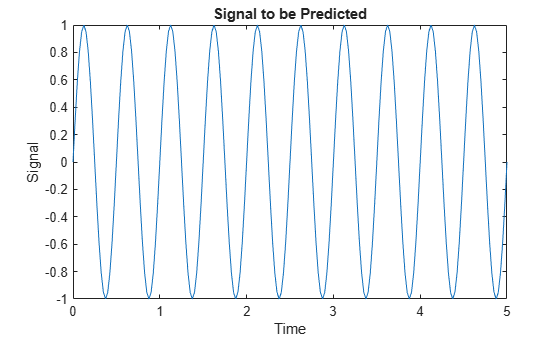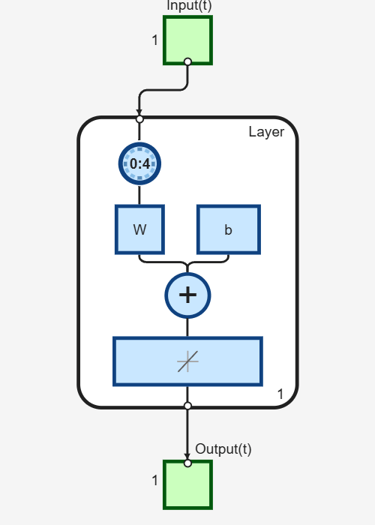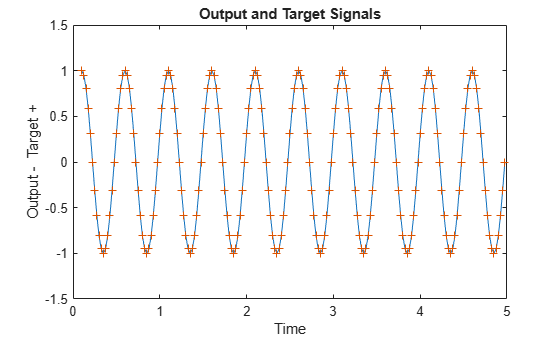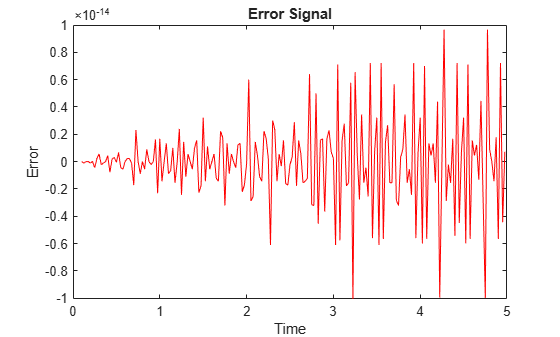Documentation

## Linear Prediction Design

This example illustrates how to design a linear neuron to predict the next value in a time series given the last five values.

### Defining a Wave Form

Here time is defined from 0 to 5 seconds in steps of 1/40 of a second.

```time = 0:0.025:5; ```

We can define a signal with respect to time.

```signal = sin(time*4*pi); plot(time,signal) xlabel('Time'); ylabel('Signal'); title('Signal to be Predicted'); ```### Setting up the Problem for a Neural Network

The signal convert is then converted to a cell array. Neural Networks represent timesteps as columns of a cell array, do distinguish them from different samples at a given time, which are represented with columns of matrices.

```signal = con2seq(signal); ```

To set up the problem we will use the first four values of the signal as initial input delay states, and the rest except for the last step as inputs.

```Xi = signal(1:4); X = signal(5:(end-1)); timex = time(5:(end-1)); ```

The targets are now defined to match the inputs, but shifted earlier by one timestep.

```T = signal(6:end); ```

### Designing the Linear Layer

The function newlind will now design a linear layer with a single neuron which predicts the next timestep of the signal given the current and four past values.

```net = newlind(X,T,Xi); view(net) ```### Testing the Linear Layer

The network can now be called like a function on the inputs and delayed states to get its time response.

```Y = net(X,Xi); ```

The output signal is plotted with the targets.

```figure plot(timex,cell2mat(Y),timex,cell2mat(T),'+') xlabel('Time'); ylabel('Output - Target +'); title('Output and Target Signals'); ```The error can also be plotted.

```figure E = cell2mat(T)-cell2mat(Y); plot(timex,E,'r') hold off xlabel('Time'); ylabel('Error'); title('Error Signal'); ```Notice how small the error is!

This example illustrated how to design a dynamic linear network which can predict a signal's next value from current and past values.

Download ebook